# MAFS.8.G.1.5

Use informal arguments to establish facts about the angle sum and exterior angle of triangles, about the angles created when parallel lines are cut by a transversal, and the angle-angle criterion for similarity of triangles. For example, arrange three copies of the same triangle so that the sum of the three angles appears to form a line, and give an argument in terms of transversals why this is so.
General Information
Subject Area: Mathematics
Domain-Subdomain: Geometry
Cluster: Level 2: Basic Application of Skills & Concepts
Cluster: Understand congruence and similarity using physical models, transparencies, or geometry software. (Major Cluster) -

Clusters should not be sorted from Major to Supporting and then taught in that order. To do so would strip the coherence of the mathematical ideas and miss the opportunity to enhance the major work of the grade with the supporting clusters.

Date of Last Rating: 02/14
Status: State Board Approved
Assessed: Yes
Test Item Specifications

• Assessment Limits :

Items must not include shapes beyond triangles

• Calculator :

Neutral

• Context :

No context

Sample Test Items (4)
• Test Item #: Sample Item 1
• Question:

What is the measure of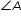, in degrees, in the figure shown?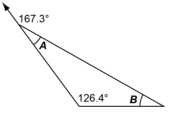• Difficulty: N/A
• Type: EE: Equation Editor

• Test Item #: Sample Item 2
• Question:

What is the measure of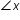, in degrees, in the figure shown?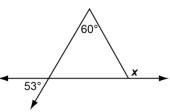• Difficulty: N/A
• Type: EE: Equation Editor

• Test Item #: Sample Item 3
• Question:

Two similar triangles are shown.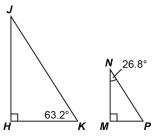What is the measure of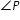, in degrees?

• Difficulty: N/A
• Type: EE: Equation Editor

• Test Item #: Sample Item 4
• Question:

A figure with parallel lines m and n is shown.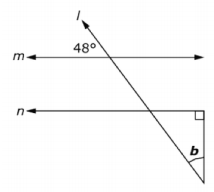What is the measure, in degrees, of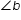?

• Difficulty: N/A
• Type: EE: Equation Editor

## Related Courses

This benchmark is part of these courses.
1205050: M/J Accelerated Mathematics Grade 7 (Specifically in versions: 2014 - 2015, 2015 - 2020, 2020 - 2022 (current), 2022 and beyond)
1205070: M/J Grade 8 Pre-Algebra (Specifically in versions: 2014 - 2015, 2015 - 2022 (current), 2022 and beyond)
1204000: M/J Foundational Skills in Mathematics 6-8 (Specifically in versions: 2014 - 2015, 2015 - 2022 (current), 2022 and beyond)
7812030: Access M/J Grade 8 Pre-Algebra (Specifically in versions: 2014 - 2015, 2015 - 2018, 2018 - 2019, 2019 and beyond (current))

## Related Access Points

Alternate version of this benchmark for students with significant cognitive disabilities.
MAFS.8.G.1.AP.5a: Use angle relationships to find the value of a missing angle.

## Related Resources

Vetted resources educators can use to teach the concepts and skills in this benchmark.

## Assessments

Sample 4 - Eighth Grade Math State Interim Assessment:

This is a State Interim Assessment for eighth grade.

Type: Assessment

Sample 3 - Eighth Grade Math State Interim Assessment:

This is a State Interim Assessment for eighth grade.

Type: Assessment

Sample 2 - Eighth Grade Math State Interim Assessment:

This is a State Interim Assessment for eighth grade.

Type: Assessment

Sample 1 - Eighth Grade Math State Interim Assessment:

This is a State Interim Assessment for eighth grade.

Type: Assessment

## Educational Software / Tool

Glossary:

This resource is an online glossary to find the meaning of math terms. Students can also use the online glossary to find words that are related to the word typed in the search box. For example: Type in "transversal" and 11 other terms will come up. Click on one of those terms and its meaning is displayed.

Type: Educational Software / Tool

## Formative Assessments

What Is the Triangle Relationship?:

Students are asked to write an informal justification of the AA Similarity Theorem.

Type: Formative Assessment

Same Side Interior Angles:

Students are asked to describe and justify the relationship between same side interior angles.

Type: Formative Assessment

Justifying the Triangle Sum Theorem:

Students are asked to provide an informal justification of the Triangle Sum Theorem.

Type: Formative Assessment

Justifying the Exterior Angle of a Triangle Theorem:

Students are asked to apply the Exterior Angle of a Triangle Theorem and provide an informal justification.

Type: Formative Assessment

Justifying Angle Relationships:

Students are asked to describe and justify the relationship between corresponding angles and alternate interior angles.

Type: Formative Assessment

## Image/Photograph

Angles (Clipart ETC):

This large collection of clipart contains images of angles that can be freely used in lesson plans, worksheets, and presentations.

Type: Image/Photograph

## Lesson Plans

Identifying Similar Triangles:

This 105-minute lesson series helps teachers assess how students reason about geometry, including how they use facts about the angle sum and exterior angles of triangles to calculate missing angles, apply angle theorems to parallel lines cut by a transversal, and interpret geometrical diagrams using mathematical properties to identify similarity of triangles. In order to complete this lesson, students will need whiteboards, pens, wipes, copies of the assessment tasks, pencils, markers, scissors, glue sticks, and poster paper.

Type: Lesson Plan

Scientific calculations from a distant planet:

Students will act as mathematicians and scientists as they use models, observations and space science concepts to perform calculations and draw inferences regarding a fictional solar system with three planets in circular orbits around a sun. Among the calculations are estimates of the size of the home planet (using a method more than 2000 years old) and the relative distances of the planets from their sun.

Type: Lesson Plan

Shape It Up:

Students will draw diagonals for different polygons, separating the polygons into triangles. Using the fact that the sum of the measures of the interior angles of a triangle is 180 degrees, and the fact the angles of the triangles are used to form the angles of the polygons, students will derive the formula for finding the sum of the measures of the angles of a polygon with n sides. Students will also learn to use this formula, along with the fact that all angles of a regular polygon are congruent, to find the measures of the angles of a regular polygon.

Type: Lesson Plan

The Ins and Outs of Polygons:

In this lesson, students will explore how to find the sum of the measures of the angles of a triangle and then be able to find the sum of the measures of the angles of other polygons. They will also be able to find the sum of the exterior angles of triangles and other polygons. Using both of these concepts, they will be able to find missing measurements.

Type: Lesson Plan

Triangles: Finding Interior Angle Measures:

In this lesson plan, students will start with a hands-on activity and then experiment with a GeoGebra-based computer model to investigate and discover the Triangle Angle Sum Theorem. Then they will use the Triangle Angle Sum Theorem to write and solve equations and find missing angle measures in a variety of examples.

Type: Lesson Plan

Special Angle Pairs Discovery Activity:

This lesson uses a discovery approach to identify the special angles formed when a set of parallel lines is cut by a transversal. During this lesson students identify the angle pair and the relationship between the angles. Students use this relationship and special angle pairs to make conjectures about which angle pairs are considered special angles.

Type: Lesson Plan

How Many Degrees?:

This lesson facilitates the discovery of a formula for the sum of the interior angles of a regular polygon. Students will draw all the diagonals from one vertex of various polygons to find how many triangles are formed. They will use this and their prior knowledge of triangles to figure out the sum of the interior angles. This will lead to the formulation of a formula for finding the sum of interior angles and the measure of one interior angle.

Type: Lesson Plan

Help me Find my Relationship!:

In this lesson, students will investigate the relationship between angles when parallel lines are cut by a transversal. Students will identify angles, find angle measures, and they will use the free application GeoGebra (see download link under Suggested Technology) to provide students with a visual representation of angles relationships.

Type: Lesson Plan

An Investigation of Angle Relationships Formed by Parallel Lines Cut by a Transversal Using GeoGebra:

In this lesson, students will discover angle relationships formed (corresponding, alternate interior, alternate exterior, same-side interior, same-side exterior) when two parallel lines are cut by a transversal. They will establish definitions and identify whether these angle pairs are supplementary or congruent.

Type: Lesson Plan

Tile Patterns I: octagons and squares:

In this task students are given a tile pattern involving congruent regular octagons and squares. They are asked to determine the interior angle measure of the octagon and verify the attributes of the square.

Find the Angle:

Use informal arguments to establish facts about the angle sum and exterior angle of triangles, about the angles created when parallel lines are cut by a transversal, and the angle-angle criterion for similarity of triangles.

Find the Missing Angle:

This task provides us with the opportunity to see how the mathematical ideas embedded in the standards and clusters mature over time. The task "Uses facts about supplementary, complementary, vertical, and adjacent angles in a multi-step problem to write and solve simple equations for an unknown angle in a figure ()" except that it requires students to know, in addition, something about parallel lines, which students will not see until 8th grade. As a result, this task is especially good at illustrating the links between related standards across grade levels.

Tile Patterns II: hexagons:

This task is ideally suited for instruction purposes where students can take their time and develop several of the Mathematical Practice standards, as the mathematical content is directly related to, but somewhat exceeds, the content of standard on sums of angles in triangles. Careful analysis of the angles requires students to construct valid arguments (MAFS.K12.MP.3.1) using abstract and quantitative reasoning (MAFS.K12.MP.2.1). Producing the picture in part (c) helps students identify a common mathematical argument repeated multiple times (MAFS.K12.MP.8.1). If students use pattern blocks in order to develop the intuition for decomposing the hexagon into triangles, then this is also an example of MAFS.K12.MP.5.1.

## Student Center Activity

Students can practice answering mathematics questions on a variety of topics. With an account, students can save their work and send it to their teacher when complete.

Type: Student Center Activity

## Tutorials

Finding Missing Angle Measures:

In this video, we find missing angle measures from a variety of examples.

Type: Tutorial

Proving congruent angles:

In this tutorial, students are asked to prove two angles congruent when given limited information. Students need to have a foundation of parallel lines, transversals and triangles before viewing this video.

Type: Tutorial

Sum of measures of triangles proof:

This video gives the proof of sum of measures of angles in a triangle. This video is beneficial for both Algebra and Geometry students.

Type: Tutorial

## MFAS Formative Assessments

Justifying Angle Relationships:

Students are asked to describe and justify the relationship between corresponding angles and alternate interior angles.

Justifying the Exterior Angle of a Triangle Theorem:

Students are asked to apply the Exterior Angle of a Triangle Theorem and provide an informal justification.

Justifying the Triangle Sum Theorem:

Students are asked to provide an informal justification of the Triangle Sum Theorem.

Same Side Interior Angles:

Students are asked to describe and justify the relationship between same side interior angles.

What Is the Triangle Relationship?:

Students are asked to write an informal justification of the AA Similarity Theorem.

## Student Resources

Vetted resources students can use to learn the concepts and skills in this benchmark.

## Educational Software / Tool

Glossary:

This resource is an online glossary to find the meaning of math terms. Students can also use the online glossary to find words that are related to the word typed in the search box. For example: Type in "transversal" and 11 other terms will come up. Click on one of those terms and its meaning is displayed.

Type: Educational Software / Tool

Tile Patterns I: octagons and squares:

In this task students are given a tile pattern involving congruent regular octagons and squares. They are asked to determine the interior angle measure of the octagon and verify the attributes of the square.

Find the Angle:

Use informal arguments to establish facts about the angle sum and exterior angle of triangles, about the angles created when parallel lines are cut by a transversal, and the angle-angle criterion for similarity of triangles.

Find the Missing Angle:

This task provides us with the opportunity to see how the mathematical ideas embedded in the standards and clusters mature over time. The task "Uses facts about supplementary, complementary, vertical, and adjacent angles in a multi-step problem to write and solve simple equations for an unknown angle in a figure ()" except that it requires students to know, in addition, something about parallel lines, which students will not see until 8th grade. As a result, this task is especially good at illustrating the links between related standards across grade levels.

Tile Patterns II: hexagons:

This task is ideally suited for instruction purposes where students can take their time and develop several of the Mathematical Practice standards, as the mathematical content is directly related to, but somewhat exceeds, the content of standard on sums of angles in triangles. Careful analysis of the angles requires students to construct valid arguments (MAFS.K12.MP.3.1) using abstract and quantitative reasoning (MAFS.K12.MP.2.1). Producing the picture in part (c) helps students identify a common mathematical argument repeated multiple times (MAFS.K12.MP.8.1). If students use pattern blocks in order to develop the intuition for decomposing the hexagon into triangles, then this is also an example of MAFS.K12.MP.5.1.

## Student Center Activity

Students can practice answering mathematics questions on a variety of topics. With an account, students can save their work and send it to their teacher when complete.

Type: Student Center Activity

## Tutorials

Finding Missing Angle Measures:

In this video, we find missing angle measures from a variety of examples.

Type: Tutorial

Proving congruent angles:

In this tutorial, students are asked to prove two angles congruent when given limited information. Students need to have a foundation of parallel lines, transversals and triangles before viewing this video.

Type: Tutorial

Sum of measures of triangles proof:

This video gives the proof of sum of measures of angles in a triangle. This video is beneficial for both Algebra and Geometry students.

Type: Tutorial

## Parent Resources

Vetted resources caregivers can use to help students learn the concepts and skills in this benchmark.

## Educational Software / Tool

Glossary:

This resource is an online glossary to find the meaning of math terms. Students can also use the online glossary to find words that are related to the word typed in the search box. For example: Type in "transversal" and 11 other terms will come up. Click on one of those terms and its meaning is displayed.

Type: Educational Software / Tool

Tile Patterns I: octagons and squares:

In this task students are given a tile pattern involving congruent regular octagons and squares. They are asked to determine the interior angle measure of the octagon and verify the attributes of the square.

Find the Angle:

Use informal arguments to establish facts about the angle sum and exterior angle of triangles, about the angles created when parallel lines are cut by a transversal, and the angle-angle criterion for similarity of triangles.